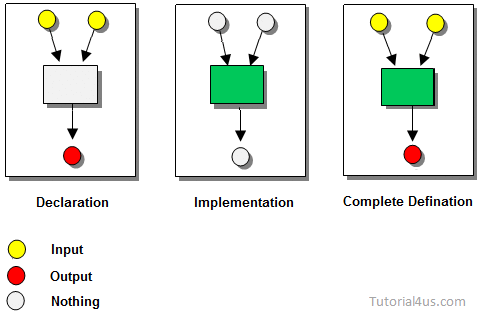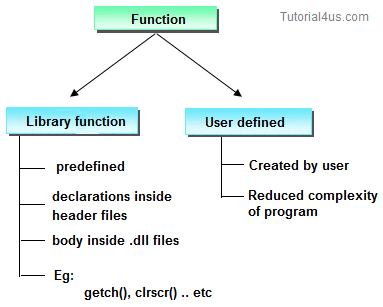# Function in C++

## Function in C++

A function is a group of statements that together perform a specific task. Every C++ program has at least one function, which is main().## Why use function ?

Function are used for divide a large code into module, due to this we can easily debug and maintain the code. For example if we write a calculator programs at that time we can write every logic in a separate function (For addition sum(), for subtraction sub()). Any function can be called many times.

• Code Re-usability
• Develop an application in module format.
• Easily to debug the program.
• Code optimization: No need to write lot of code.

## Type of Function

There are two type of function in C++ Language. They are;

• Library function or pre-define function.
• User defined function.## Library function

Library functions are those which are predefined in C++ compiler. The implementation part of pre-defined functions is available in library files that are .lib/.obj files. .lib or .obj files are contained pre-compiled code. printf(), scanf(), clrscr(), pow() etc. are pre-defined functions.

## Limitations of Library function

• All predefined function are contained limited task only that is for what purpose function is designed for same purpose it should be used.
• As a programmer we do not having any controls on predefined function implementation part is there in machine readable format.
• In implementation whenever a predefined function is not supporting user requirement then go for user defined function.

## User defined function

These functions are created by programmer according to their requirement for example suppose you want to create a function for add two number then you create a function with name sum() this type of function is called user defined function.

## Defining a function.

Defining of function is nothing but give body of function that means write logic inside function body.

## Syntax

```
return_type  function_name(parameter)
{
function body;
}
```
• Return type: A function may return a value. The return_type is the data type of the value the function returns.Return type parameters and returns statement are optional.
• Function name: Function name is the name of function it is decided by programmer or you.
• Parameters: This is a value which is pass in function at the time of calling of function A parameter is like a placeholder. It is optional.
• Function body: Function body is the collection of statements.

## Function Declarations

A function declaration is the process of tells the compiler about a function name. The actual body of the function can be defined separately.

## Syntax

```return_type  function_name(parameter);
```

Note: At the time of function declaration function must be terminated with ';'.

## calling a function.

When we call any function control goes to function body and execute entire code. For call any function just write name of function and if any parameter is required then pass parameter.

## Syntax

```function_name();
or
variable=function_name(argument);
```

Note: At the time of function calling function must be terminated with ';'.

## Example of Function in C++

```
#include<iostream.h>
#include<conio.h>

void sum(); // declaring a function
int a=10,b=20, c;

void sum()  // defining function
{
c=a+b;
cout<<"Sum: "<<c;
}
void main()
{
clrsct();
sum();  // calling function
}
```

```
Sum: 30
```